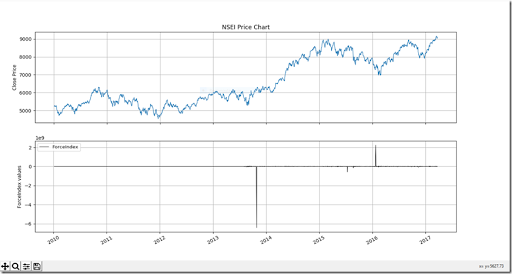### Force Index Technical Indicators in Python to Measure Buying and Selling Pressure .### The force index (FI) is an indicator used in technical analysis

to illustrate how strong the actual buying or selling pressure is. High positive values mean there is a strong rising trend, and low values signify a strong downward trend.
The FI is calculated by multiplying the difference between the last and previous closing prices by the volume of the commodity, yielding a momentum scaled by the volume. The strength of the force is determined by a larger price change or by a larger volume.
The world of trading and technical analysis is continually developing new ways to make the most of our most basic trading tools and indicators; Dr Alexander Elder is one of the contributors to a newer generation of technical indicators. In this article, we look at his force index, which is an oscillator that measures the force, or the power, of bulls

behind particular market rallies and of bears behind every decline.
The three key components of the force index are the direction of price change, the extent of the price change and the trading volume. When the force index is used in conjunction with a moving averag (MA), the resulting figure can accurately measure significant changes in the power of bulls and bears. In this way, Dr Elder has taken an extremely useful solitary indicator, the moving average, and combined it with his force index for even greater predictive success.The Calculation
The force index is calculated by subtracting yesterday's close from today's close and multiplying the result by today's volume. If closing prices are higher today than yesterday, the force is positive. If closing prices are lower than yesterday's, the force is negative. The strength of the force is determined either by a larger change in price or a larger volume; either situation can independently influence the value and the change in force index.
The raw value of force index is plotted as a histogram, with the center line set to zero. A higher market will result in a positive force index, plotted above the center line; a lower market points to a negative force index, below the center line. An unchanged market will return a force index directly on the zero line. The raw line that is plotted over the day-to-day on the histogram forms a jaggedness and the moving average smooths the line. Therefore, at minimum, you'll want to use a two-day exponential moving average
(EMA) for the appropriate level of smoothing.
Interpreting the Force Index
In general, traders will want to buy when the two-day EMA of force index is negative and sell when it is positive. These traders, however, should always keep in mind the overarching principle of trading in the direction of the 13-day EMA of prices. The 13-day EMA of force index is a longer-term indicator, and, when it crosses above the centerline, the bulls are exerting the greatest force. When it is negative, the bears have control of the market. Of particular importance are divergences between a 13-day EMA of force index and prices, which correspond with precise points, indicating crucial turning points of the market.
As indicated by closing prices, the difference between yesterday and today's close gives the degree of the day-to-day victory of either the bulls or the bears. Similarly, volume is added into the calculation to give a greater sense of the degree of bulls or bears' victories. Volume also indicates the level of momentum in the market, as propelled by the power of either bulls or bears. Force index is one of the best indicators for combining both price and volume into a single readable figure. When force index hits a new high, a given uptrend is likely to continue. When force index hits a new low, the bears have greater strength and the downtrend will usually sustain itself.
A flattening force index is also an important situational circumstance for traders. A flattening force index means that the observed change in prices is not supported by either rising or declining volume and that the trend is about to reverse. On the opposite side of the matter, a flattening force index could indicate a trend reversal, if a high volume corresponds with only a small move in prices.
So, this is the basic manner in which force index can be used alone, or in conjunction with a moving average, to identify whether bulls or bears have control of the market. When volume is considered, an accurate sense of the market's momentum may also be quickly garnered.
The Bottom LineForce index is an indicator that can be further refined, according to whether a trader wishes to adopt a short-term or a longer-term perspective. The two-day EMA of force index mentioned above supports a whole host of additional trading rules that offer precise trend indicators for exact trading situations. On an intermediate basis, a 13-day EMA of force index can point to the likelihood of sustained rallies or longer-term market declines, thereby generating trading rules for longer-term decision making.

## Computing Force index(1) and Force index(15) period

:

### Code Input :

``````
#Technical indicator Force index
import pandas as pd
import pandas_datareader.data as web
import matplotlib.pyplot as plt

def ForceIndex(data,ndays):
ForceIndex=pd.Series(data['Close'].diff(ndays)* data['Volume'],name='ForceIndex')
data=data.join(ForceIndex)
return data

data=pd.DataFrame(data)

n=1
NSEI_ForceIndex = ForceIndex(data,n)
ForceIndex = NSEI_ForceIndex['ForceIndex']

fig=plt.figure(figsize=(7,5))
ax.set_xticklabels([])
plt.plot(data['Close'],lw=1)
plt.title('NSEI Price Chart')
plt.ylabel('Close Price')
plt.grid(True)